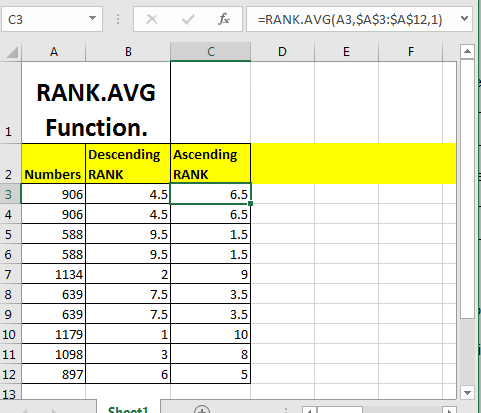# Excel RANK.AVG Function

The RANK.AVG function in excel ranks a number in a reference list of numbers. When there are two or more equal numbers are found, it calculates the average rank and assigns that rank to each clashing numbers.#### Syntax of RANK.AVG Function

=RANK.AVG(number,ref_range,[order])

Number: The number that you want to rank
Ref_Range: The range in which you want to rank. The number must be in the ref_range.

[Order]: This argument is optional. 1 is for ascending ranking and 0 for descending. Ascending ranking means that higher number will get lover ranking. Descending ranking means higher number will get higher ranking. By default it is Descending.

#### RANK.AVG FUNCTION EXAMPLE

So to rank each number in range A3:A12 in descending order we can write this RANK.AVG formula.

=RANK.AVG(A3,\$A\$3:\$A\$12)

Similarly to rank each number in range A3:A12 in ascending order write this RANK.AVG formula.

=RANK.AVG(A3,\$A\$3:\$A\$12,1)

1 commands excel to rank in ascending order.

The best thing about the RANK.AVG function that you don’t need to sort the data to rank.

Since Excel 2010 till now RANK.AVG function is used to rank numbers with average ranking in excel.

Related Articles:

Excel RANK.EQ Function

Popular Articles:

50 Excel Shortcuts to Increase Your Productivity

How to use the VLOOKUP Function in Excel

How to use the COUNTIF function in Excel 2016

How to Use SUMIF Function in Excel

Terms and Conditions of use

The applications/code on this site are distributed as is and without warranties or liability. In no event shall the owner of the copyrights, or the authors of the applications/code be liable for any loss of profit, any problems or any damage resulting from the use or evaluation of the applications/code.